• 分布式训练之数据并行 1. 背景 ​ 在深度学习的发展历程中，通过改变神经网络的结构，可以取得比较明显的精度。但是，随着神经网络结构设计技术不断成熟，想通过优化神经网络结构来打破模型的精度瓶颈遇到了很大的...

分布式训练之数据并行

1. 背景

​ 在深度学习的发展历程中，通过改变神经网络的结构，可以取得比较明显的精度。但是，随着神经网络结构设计技术不断成熟，想通过优化神经网络结构来打破模型的精度瓶颈遇到了很大的挑战。

​ 根据一些研究表明，通过增大数据规模和模型规模，可以进一步提升模型精度。但是，这也意味着训练时间会变长，所以可以增加计算资源，通过分布式训练来缩短训练时间，将单卡的负载拆到多卡上。

数据并行（Data Parallelism） 通过修改Sampler切分输入，每张卡只需要处理一部分数据；

模型并行（Model Parallelism） 通过修改层内的计算方式，将单层的计算负载和显存负载切分到多张卡上；

流水并行（Pipeline Parallelism） 通过将不同的层放到不同的卡上，进而将计算负载和显存负载切分到多张卡上。

2. 数据并行

​ 在深度学习中，数据集越来越大，我们很难一次性将所有的数据集加载到内存中，所以我们会使用随机梯度下降法，即每次只用一个batch的数据进行训练，对损失函数求导，得到每个参数的梯度。例如，在一个有51200个样本的数据集中，每次只取512个样本进行梯度的计算（batch_size=512），所以要将数据集迭代一遍需要100次，训练时间非常长。

​ 为了加快模型的训练，可以使用数据并行的分布式训练方法。将一个大批次分割为很多小批次，使用很多节点进行计算，在每个节点上计算一个小批次，对若干个节点的梯度进行汇总后再加权平均，最终求和就得到了最终的大批次的梯度结果。∂ L ∂ w = ∂ [ 1 n ∑ i = 1 n f ( x ( i ) , y ( i ) ) ] ∂ w = m 1 n ∂ l 1 ∂ w + m 2 n ∂ l 2 ∂ w + ⋯ + m k n ∂ l k ∂ w \frac{\partial L}{\partial w}=\frac{\partial[\frac{1}{n}\sum_{i=1}^{n}f(x^{(i)},y^{(i)})]}{\partial w}=\frac{m_1}{n}\frac{\partial l_1}{\partial w}+\frac{m_2}{n}\frac{\partial l_2}{\partial w}+\dots+\frac{m_k}{n}\frac{\partial l_k}{\partial w}
​ 在上面这个公式中， w w 是模型的参数， ∂ L ∂ w \frac{\partial L}{\partial w} 是在batch_size为n的情况下计算得到的真实梯度，共有 k k 个节点，在第 j j 个节点上有 m j m_j 个数据， ∂ l j ∂ w \frac{\partial l_{j}}{\partial w} 是在该节点上计算得到的精度， m 1 + m 2 + ⋯ + m k = n m_1+m_2+\dots+m_k=n n n 个样本数据被拆分到了 k k 个姐弟节点上）， x ( i ) x^{(i)} y ( i ) y^{(i)} 是样本数据 i i 的特征和标签，对于样本数据 i i f ( x ( i ) , y ( i ) ) f(x^{(i)},y^{(i)}) 是前向传播的损失函数。

​ 公式表达的含义是在不同的节点上分别对一部分数据进行梯度的计算，将各个GPU的梯度进行汇总后，计算其加权平均值

​ 如果每个节点上的数据量是平分的，则
∂ L ∂ w = 1 k [ ∂ l 1 ∂ w + ∂ l 2 ∂ w + ⋯ + ∂ l k ∂ w ] \frac{\partial L}{\partial w}=\frac{1}{k}[\frac{\partial l_1}{\partial w}+\frac{\partial l_2}{\partial w}+\dots+\frac{\partial l_k}{\partial w}]
对于每个节点，我们使用相同的模型参数进行前向传播；我们将大批次的数据分割成很多个小批次的数据，发送到不同的节点上，每个节点都正常计算其梯度，计算完返回其梯度计算结果到主节点。这一步是异步的，因为每个节点的速度都可能稍有差别，一旦得到了所有的梯度，就可以计算这些梯度的加权平均，并使用这个加权平均的梯度去更新整个模型的参数。

3. PyTorch数据并行实现

DistributedDataParallel(DDP)在模型层面实现了数据并行。使用DDP的应用程序应该派生多个进程，并为每个进程创建一个DDP实例。它使用torch.distributed程序包中的通信集合来同步梯度和缓冲区。更具体地说，DDP给model.parameters()的每个参数都注册了一个自动的hook，在反向传播中相应的梯度被计算出来时，hook将会fire。然后，DDP使用一个信号来引发跨进程间的梯度同步。

​ 推荐使用DDP的方法是给每个复制的模型派生一个进程，一个复制的模型可以跨多台设备。DDP进程可以放在相同的机器，或者跨多台机器，但是GPU设备不能跨进程分享。

3.1 DataParallel和DistributedDataParallel之间的比较

​ 在深入探讨前，先澄清一下，为什么增加了复杂度，但还是要使用DistributedDataParallel而不是DataParallel

• 首先，DataParallel是单进程、多线程的，只能工作在一台机器上。但是，DistributedDataParallel是多进程，可以在单台或多台机器上使用。DataParallel在单台机器上甚至比DistributedDataParallel要慢。
• 如果模型太大而无法放到一张GPU上，就必须使用模型并行来将其分到多张GPU上。DistributedDataParallel支持模型并行，但是DataParallel暂时还不支持。当DDP和模型并行相结合时，每个DDP进行将使用模型并行，并且所有的进程将一起使用数据并行。

3.2 基本用例

​ 为了创建DDP模块，首先要合理地设置进程组，详见Writing Distributed Applications with PyTorch

import os
import sys
import tempfile
import torch
import torch.distributed as dist
import torch.nn as nn
import torch.optim as optim
import torch.multiprocessing as mp

from torch.nn.parallel import DistributedDataParallel as DDP

def setup(rank, world_size):
os.environ['MASTER_PORT'] = '12355'

# initialize the process group
dist.init_process_group("gloo", rank=rank, world_size=world_size)

def cleanup():
dist.destroy_process_group()

​ 现在，可以创建一个toy module，用DDP进行包装，并喂给它一些假的输入数据。当DDP把model states从rank 0进程传播到DDP构造器的所有其他进程中时，不需要不同的DDP进程的模型初始化参数值不同。

class ToyModel(nn.Module):
def __init__(self):
super(ToyModel, self).__init__()
self.net1 = nn.Linear(10, 10)
self.relu = nn.ReLU()
self.net2 = nn.Linear(10, 5)

def forward(self, x):
return self.net2(self.relu(self.net1(x)))

def demo_basic(rank, world_size):
print(f"Running basic DDP example on rank {rank}.")
setup(rank, world_size)

# create model and move it to GPU with id rank
model = ToyModel().to(rank)
ddp_model = DDP(model, device_ids=[rank])

loss_fn = nn.MSELoss()
optimizer = optim.SGD(ddp_model.parameters(), lr=0.001)

outputs = ddp_model(torch.randn(20, 10))
labels = torch.randn(20, 5).to(rank)
loss_fn(outputs, labels).backward()
optimizer.step()

cleanup()

def run_demo(demo_fn, world_size):
mp.spawn(demo_fn,
args=(world_size,),
nprocs=world_size,
join=True)

4. 参考资料

大规模训练系列之技术挑战

分布式深度学习简介：数据并行和模型并行

展开全文分布式 深度学习 神经网络 人工智能
• 数据并行与模型并行 为了加快模型的训练，可以使用分布式计算的思路，把这个大批次分割为很多小批次，使用多个节点进行计算，在每个节点上计算一个小批次，对若干个节点的梯度进行汇总后再加权平均，最终求和就得到...

数据并行与模型并行
为了加快模型的训练，可以使用分布式计算的思路，把这个大批次分割为很多小批次，使用多个节点进行计算，在每个节点上计算一个小批次，对若干个节点的梯度进行汇总后再加权平均，最终求和就得到了最终的大批次的梯度结果。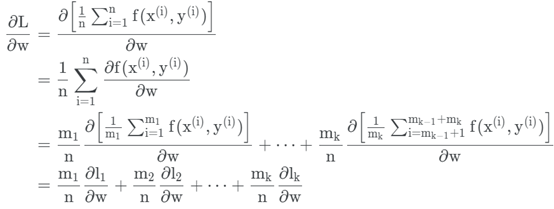在上面这个公式中：w是模型的参数；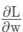是采用batch_size = n计算得到的真实梯度。这个公式想要证明的是，可以在不同的节点上分别对n的一部分进行梯度的计算，将各个GPU的梯度进行汇总后的加权平均值。公式中最后一行中，在第k个节点有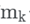个数据，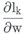是该节点上计算得到梯度。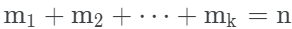，n个样本数据被分拆到了多个节点上。
其中，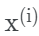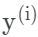是样本数据i的特征和标签；对于样本数据i，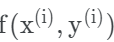是前向传播的损失函数。
如果对每个节点上的数据量平分，有：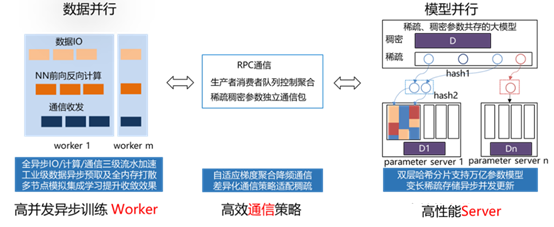图1. 传统参数服务器工作流程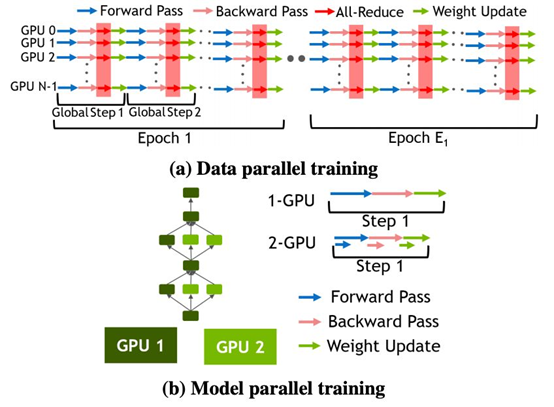图 2. 不同的训练并行化策略，2(a) 展示了数据并行化训练，2(b) 展示了模型并行化训练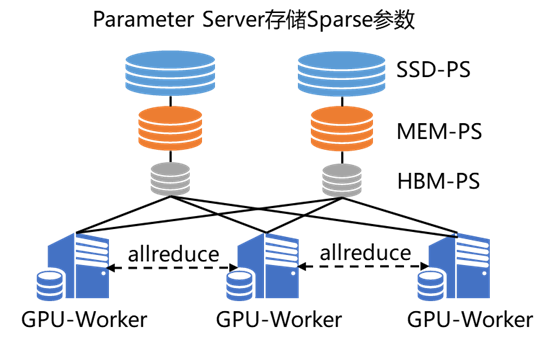图3. 纯GPU参数服务器工作流程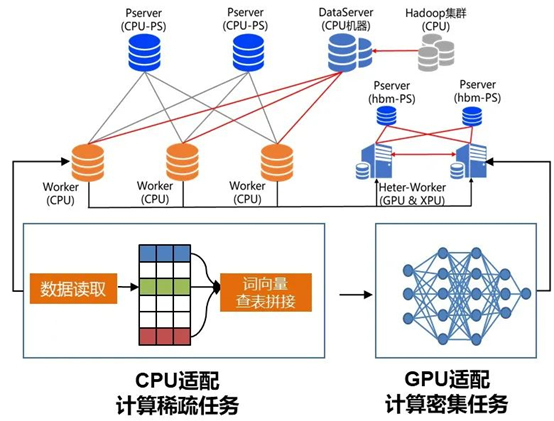图4. 异构参数服务器示意图

展开全文• 单卡多级的模型训练，即并行训练，可分为数据并行和模型并行两种. 数据并行是指，多张 GPUs 使用相同的模型副本，但采用不同 batch 的数据进行训练. 模型并行是指，多张 GPUs 使用同一 batch 的数据，分别训练模型的...

单卡多级的模型训练，即并行训练，可分为数据并行和模型并行两种.

数据并行是指，多张 GPUs 使用相同的模型副本，但采用不同 batch 的数据进行训练.

模型并行是指，多张 GPUs 使用同一 batch 的数据，分别训练模型的不同部分.

如图：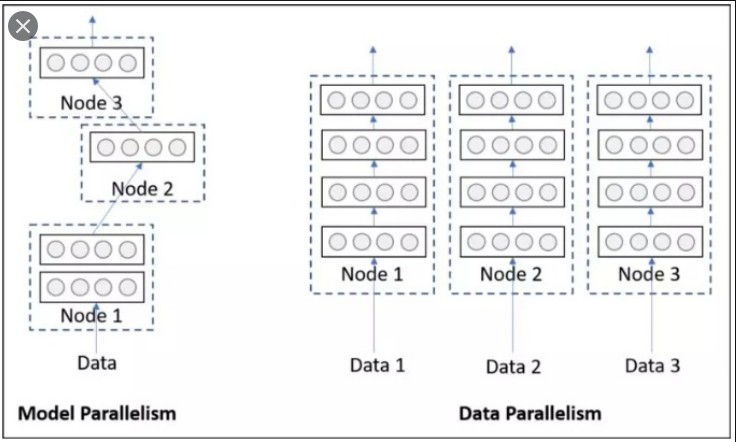1. DataParallel 简述

pytorch 默认只用一个 GPU，但采用 DataParallel很便于使用多 GPUs 的.
import os
os.environ["CUDA_VISIBLE_DEVICES"] = "1,2"

# 注: 多卡训练时，默认将 model 和 data 先保存到 id:0 的卡上(这里是第1块卡)
#     然后 model 的参数再复制共享到其他卡上；
#     data 也会平分为 subbatch 到其他卡上.
# 故：一般第一张卡显存占用多点.
device = torch.device("cuda:0")

# 1.将模型放到 GPU 上
device_ids = [0, 1] #必须从零开始(这里0表示第1块卡，1表示第2块卡.)
model = nn.DataParallel(model, device_ids=device_ids)
model.to(device)

# 2.将id:0 卡数据平分到其他卡.
data.to(device)

注：data.to(device) 返回 data 在 GPU 上的一份副本，而不是重写 data.

2. nn.DataParallel 函数

class torch.nn.DataParallel(module, device_ids=None, output_device=None, dim=0)

 - module ：待进行并行的模块.

 - device_ids : GPU 列表，其值可以是 torch.device 类型，也可以是 int list. 默认使用全部 GPUs.

 - output_device : GPUID 或 torch.device. 指定输出的 GPU，默认为第一个，即 device_ids.

3. DataParallel 原理

pytorch 单机多卡的基本原理如下.

假设读入一个 batch 的数据，其大小为 [30, 5, 2]，假设采用三张 GPUs，其运行过程大致为：

 - 将模型放到主 GPU 上，一般为 cuda:0;

 - 把模型同步到 3 张 GPUs 上;

 - 将总输入 batch 的数据平分为 3 份，这里每一份大小为 [10, 5, 2]；

 - 依次分别作为每个副本模型的输入；

 - 每个副本模型分别独立进行前向计算，假设为 [4, 5, 2];

 - 从 3 个 GPUs 中收集分别计算后的结果，并按照次序拼接，即 [12, 5, 2]，计算 loss;

 - 更新梯度，后向计算.

模型同步 - 数据分发 - 分别前向计算 - loss 计算 - 梯度反传.

4. 官方示例

https://pytorch.org/tutorials/beginner/blitz/data_parallel_tutorial.html

import torch
import torch.nn as nn

input_size = 5
output_size = 2
batch_size = 30
data_size = 100

# Device
device = torch.device("cuda:0" if torch.cuda.is_available() else "cpu")

# Dummy Dataset
class RandomDataset(Dataset):
def __init__(self, size, length):
self.len = length
self.data = torch.randn(length, size)

def __getitem__(self, index):
return self.data[index]

def __len__(self):
return self.len
batch_size=batch_size, shuffle=True)

# demo model - linear operation
class Model(nn.Module):
def __init__(self, input_size, output_size):
super(Model, self).__init__()
self.fc = nn.Linear(input_size, output_size)

def forward(self, input):
output = self.fc(input)
print("\tIn Model: input size", input.size(),
"output size", output.size())
return output

# 单机多卡
# 1. 采用 nn.DataParallel 封装模型；
# 2. 采用 mode.to(device) 将模型放于多块 GPUs 上.
model = Model(input_size, output_size)
if torch.cuda.device_count() > 1:
print("Let's use", torch.cuda.device_count(), "GPUs!")
# dim = 0 [30, xxx] -> [10, ...], [10, ...], [10, ...] on 3 GPUs
model = nn.DataParallel(model)
model.to(device)

# 模型运行
# 查看 input tensors 和 output tensors 的sizes.
input = data.to(device)
output = model(input)
print("Outside: input size", input.size(),
"output_size", output.size())

 - 对于没有 GPU 或者只有一个 GPU时，当batchsize 为 30 个 inputs 和 30 个 outputs 时，模型也会得到 30 个 inputs 和 30 个 outputs.

 - 两块 GPUs 的输出如：

# on 2 GPUs
Let's use 2 GPUs!
In Model: input size torch.Size([15, 5]) output size torch.Size([15, 2])
In Model: input size torch.Size([15, 5]) output size torch.Size([15, 2])
Outside: input size torch.Size([30, 5]) output_size torch.Size([30, 2])
In Model: input size torch.Size([15, 5]) output size torch.Size([15, 2])
In Model: input size torch.Size([15, 5]) output size torch.Size([15, 2])
Outside: input size torch.Size([30, 5]) output_size torch.Size([30, 2])
In Model: input size torch.Size([15, 5]) output size torch.Size([15, 2])
In Model: input size torch.Size([15, 5]) output size torch.Size([15, 2])
Outside: input size torch.Size([30, 5]) output_size torch.Size([30, 2])
In Model: input size torch.Size([5, 5]) output size torch.Size([5, 2])
In Model: input size torch.Size([5, 5]) output size torch.Size([5, 2])
Outside: input size torch.Size([10, 5]) output_size torch.Size([10, 2])

 - 三块 GPUs 的输出如：

Let's use 3 GPUs!
In Model: input size torch.Size([10, 5]) output size torch.Size([10, 2])
In Model: input size torch.Size([10, 5]) output size torch.Size([10, 2])
In Model: input size torch.Size([10, 5]) output size torch.Size([10, 2])
Outside: input size torch.Size([30, 5]) output_size torch.Size([30, 2])
In Model: input size torch.Size([10, 5]) output size torch.Size([10, 2])
In Model: input size torch.Size([10, 5]) output size torch.Size([10, 2])
In Model: input size torch.Size([10, 5]) output size torch.Size([10, 2])
Outside: input size torch.Size([30, 5]) output_size torch.Size([30, 2])
In Model: input size torch.Size([10, 5]) output size torch.Size([10, 2])
In Model: input size torch.Size([10, 5]) output size torch.Size([10, 2])
In Model: input size torch.Size([10, 5]) output size torch.Size([10, 2])
Outside: input size torch.Size([30, 5]) output_size torch.Size([30, 2])
In Model: input size torch.Size([4, 5]) output size torch.Size([4, 2])
In Model: input size torch.Size([4, 5]) output size torch.Size([4, 2])
In Model: input size torch.Size([2, 5]) output size torch.Size([2, 2])
Outside: input size torch.Size([10, 5]) output_size torch.Size([10, 2])

 - 8 块 GPUs 的输出如：

Let's use 8 GPUs!
In Model: input size torch.Size([4, 5]) output size torch.Size([4, 2])
In Model: input size torch.Size([4, 5]) output size torch.Size([4, 2])
In Model: input size torch.Size([2, 5]) output size torch.Size([2, 2])
In Model: input size torch.Size([4, 5]) output size torch.Size([4, 2])
In Model: input size torch.Size([4, 5]) output size torch.Size([4, 2])
In Model: input size torch.Size([4, 5]) output size torch.Size([4, 2])
In Model: input size torch.Size([4, 5]) output size torch.Size([4, 2])
In Model: input size torch.Size([4, 5]) output size torch.Size([4, 2])
Outside: input size torch.Size([30, 5]) output_size torch.Size([30, 2])
In Model: input size torch.Size([4, 5]) output size torch.Size([4, 2])
In Model: input size torch.Size([4, 5]) output size torch.Size([4, 2])
In Model: input size torch.Size([4, 5]) output size torch.Size([4, 2])
In Model: input size torch.Size([4, 5]) output size torch.Size([4, 2])
In Model: input size torch.Size([4, 5]) output size torch.Size([4, 2])
In Model: input size torch.Size([4, 5]) output size torch.Size([4, 2])
In Model: input size torch.Size([2, 5]) output size torch.Size([2, 2])
In Model: input size torch.Size([4, 5]) output size torch.Size([4, 2])
Outside: input size torch.Size([30, 5]) output_size torch.Size([30, 2])
In Model: input size torch.Size([4, 5]) output size torch.Size([4, 2])
In Model: input size torch.Size([4, 5]) output size torch.Size([4, 2])
In Model: input size torch.Size([4, 5]) output size torch.Size([4, 2])
In Model: input size torch.Size([4, 5]) output size torch.Size([4, 2])
In Model: input size torch.Size([4, 5]) output size torch.Size([4, 2])
In Model: input size torch.Size([4, 5]) output size torch.Size([4, 2])
In Model: input size torch.Size([4, 5]) output size torch.Size([4, 2])
In Model: input size torch.Size([2, 5]) output size torch.Size([2, 2])
Outside: input size torch.Size([30, 5]) output_size torch.Size([30, 2])
In Model: input size torch.Size([2, 5]) output size torch.Size([2, 2])
In Model: input size torch.Size([2, 5]) output size torch.Size([2, 2])
In Model: input size torch.Size([2, 5]) output size torch.Size([2, 2])
In Model: input size torch.Size([2, 5]) output size torch.Size([2, 2])
In Model: input size torch.Size([2, 5]) output size torch.Size([2, 2])
Outside: input size torch.Size([10, 5]) output_size torch.Size([10, 2])

5. DistributedDataPrallel

pytorch官网建议使用DistributedDataParallel来代替DataParallel, 据说是因为DistributedDataParallelDataParallel运行的更快, 然后显存分屏的更加均衡. 而且DistributedDataParallel功能更加强悍, 例如分布式的模型(一个模型太大, 以至于无法放到一个GPU上运行, 需要分开到多个GPU上面执行). 只有DistributedDataParallel支持分布式的模型像单机模型那样可以进行多机多卡的运算.

分布式训练与单机多卡的区别：

 - 模型部分使用DistributedDataParallel.

主要代码如：

from torch.utils.data.distributed import DistributedSampler
from torch.nn.parallel import DistributedDataParallel

RANK = int(os.environ['SLURM_PROCID'])  # 进程序号，用于进程间通信
LOCAL_RANK = int(os.environ['SLURM_LOCALID']) # 本地设备序号，用于设备分配.
GPU_NUM = int(os.environ['SLURM_NTASKS'])     # 使用的 GPU 总数.
IP = os.environ['SLURM_STEP_NODELIST'] #进程节点 IP 信息.
BATCH_SIZE = 16  # 单张 GPU 的大小.

def dist_init(host_addr, rank, local_rank, world_size, port=23456):
rank=rank, world_size=world_size)
torch.cuda.set_device(local_rank)
assert torch.distributed.is_initialized()

if __name__ == '__main__':
dist_init(IP, RANK, LOCAL_RANK, GPU_NUM)

# DataSet
datasampler = DistributedSampler(dataset, num_replicas=GPU_NUM, rank=RANK)

# model
model = DistributedDataPrallel(model,
device_ids=[LOCAL_RANK],
output_device=LOCAL_RANK)

6. Github - 不同加速库使用示例(单机多卡)

使用 PyTorch 编写了不同加速库在 ImageNet 上的使用示例(单机多卡).

 - nn.DataParallel 简单方便的 nn.DataParallel

 - torch.distributed 使用 torch.distributed 加速并行训练

 - torch.multiprocessing 使用 torch.multiprocessing 取代启动器

 - apex 使用 apex 再加速

 - horovod horovod 的优雅实现

这里记录了使用 4 块 Tesla V100-PICE 在 ImageNet 进行了运行时间的测试，测试结果发现 Apex 的加速效果最好，但与 Horovod/Distributed 差别不大，平时可以直接使用内置的 Distributed. Dataparallel 较慢，不推荐使用.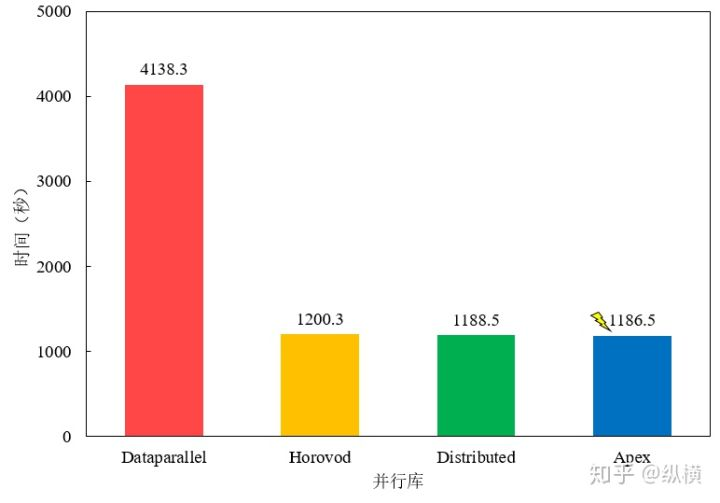参考资料

展开全文• 数据并行 由于训练数据集太大，而无法一次将其全部载入内存。因此将数据集分为N份，分别装载到N个GPU节点中去进行梯度求导，然后将所有节点的求导结果进行加权平均，再sync update给所有节点（对于每个节点来说，...

数据并行

由于训练数据集太大，而无法一次将其全部载入内存。因此将数据集分为N份，分别装载到N个GPU节点中去进行梯度求导，然后将所有节点的求导结果进行加权平均，再sync update给所有节点（对于每个节点来说，我们使用相同的模型参数进行前向传播。）。然后继续进行下一步的训练，直到模型收敛或者提前停止训练。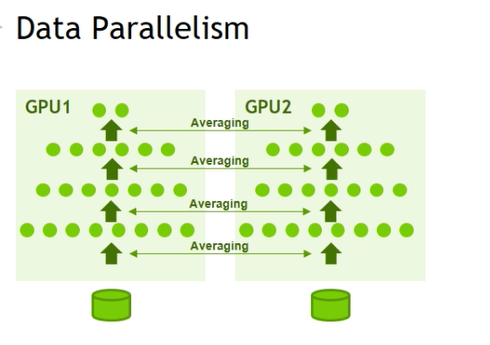如上图所示，GPU1和GPU2上都装载有完整的模型，但是它们使用不同的子数据集Batch进行训练，每次每个节点的推导结果进行加权平均然后sync到所有的GPU节点上，再继续下一步迭代。

模型并行

由于模型太大（层数太深，参数太多），而无法将整个模型载入一个GPU。因此将模型分为N个部分，分别加载到不同的N个GPU节点中。例如模型有1000层，使用10块GPU，GPU0装载模型的前1000层，以此类推，GPU9装载模型的最后1000层。在训练的时候，GPU0先进行前向传播，其结果传给GPU1，然后GPU1接着进行前向传播，以此类推，直到GPU9拿到GPU8给的结果进行反向传播。反向传播过程与前向传播过程类似，就是每个GPU节点必须等待前一个节点的结果，才能进行运算。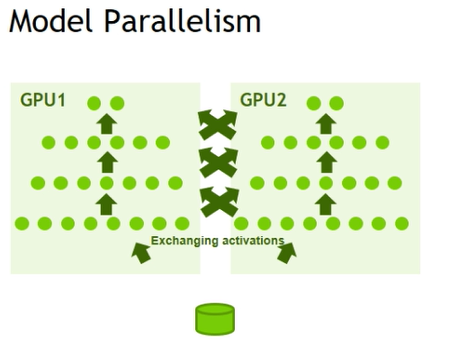如上图所示，整个模型被分为两部分，分别装载到GPU1和GPU2上。模型的分配方式可以自定义，这里是相邻层在两个GPU上，比如，GPU1上是第0，2，4等层，GPU2是第1，3，5等层。

当GPU1上的第0层前向传播得到结果，则将其传给GPU2的第1层；GPU2的第1层使用GPU0的第0层的结果进行前向传播，并将结果传给GPU1的第2层。以此类推。反向传播过程类似。

问题

哪种并行训练方式更好呢？

答案是，要结合实际情况来看。如果模型过大，当然只能使用模型并行的训练方式。

这里想强调一点的是，如果使用数据并行进行模型的并行训练，那么一定要选用DistributedDataParallel (DDP)而不是DataParallel (DP)。（在Pytorch中有这两种多GPU的数据并行训练模式）

原因是DDP的训练更快，数据传输带来的消耗更少。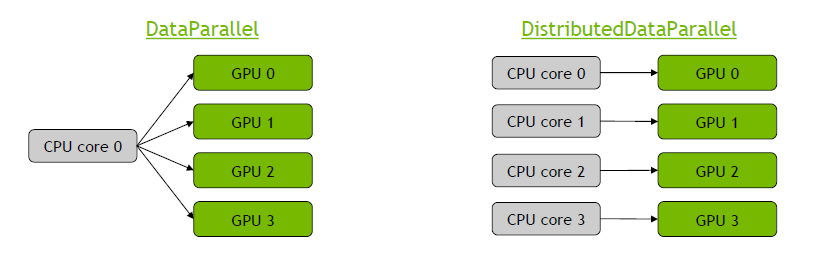DP训练模式下，所有GPU由一个CPU进程控制，模型会被加载到每一块GPU，一旦梯度在GPU0上完成了加权平均，更新后的梯度将被sync到其他所有GPU上。模型的每一层训练都是这样，那么就会产生很多GPU之间的数据传输。并且DP模式只能用在一个node（一个node上最多8块GPU）上。

DDP在每个GPU上（在其自己的进程中）创建模型的孤立副本，并使该GPU仅可使用一部分数据。 然后就像进行N次独立模型训练一样，只是每个模型都会计算梯度，它们都会在模型之间同步梯度……这意味着我们在每批处理中仅一次在GPU之间传输数据。可用于多node的分布式训练。

展开全文深度学习 人工智能
• 本文内容是如果使用多个GPU并行运算。 代码 import torch import torch.nn as nn from torch.utils.data import Dataset, DataLoader input_size = 5 output_size = 2 batch_size = 30 data_size = 100 #设备 ...神经网络 深度学习 机器学习 python pytorch
• 第四章 数据并行执行模型 《大规模并行处理器编程实战》学习，其他章节关注专栏 CUDA C 线程组成线程块，线程块组成线程网格，线程网格就是kernel。一个kernel中的所有线程都执行相同的代码，区别在于不同的线程...
• 文章目录数据并行 单机多卡训练，即并行训练。并行训练又分为数据并行 (Data Parallelism) 和模型并行两种。 数据并行指的是，多张 GPU 使用相同的模型副本，但是使用不同的数据批进行训练。而模型并行指的是，多张...深度学习
• 撰文 | 袁进辉GShard的论文最早于2020.6.30放在arXiv上，包括《GShard: Scaling Giant Models with Conditional Computa...
• 每个 GPU 在单独的线程上将针对各自的输入数据独立并行地进行 forward 计算。然后在主GPU上收集网络输出，并通过将网络输出与批次中每个元素的真实数据标签进行比较来计算损失函数值。接下来，损失值分散给各个GPU，...深度学习 pytorch
• 数据并行化是指将数据分成块，为每块数据分配单独的处理单元。《java8函数式编程》书中拿马拉车那个例子打比方，就像从车里取出一些货物，放到另一辆车上，两辆马车都沿着同样的路径到达目的地，书中画的图也是很...java 并行化
• DistributedDataParallel， 多进程，支持数据并行、模型并行，支持单机多卡、多机多卡；进程间仅传递参数，运行效率高于DataParallel 下面是一个文本分类的完整示例 import os import time import torch import ...python
• R语言栅格数据并行计算，HDF批量转TIFF 昨天讲了GLASS数据的处理：全球陆表特征参量数据产品GLASS数据批量下载、裁剪、格式转换 多波段HDF数据读取和Geotiff转换方法 今天我发现GLASS数据有的HDF里面有两个波段，...r语言
• 利用多个空闲GPU，提高模型训练推理速度 import torch from gpuinfo import GPUInfo # 查询空闲GPU gpu_ids = GPUInfo.check_empty() ...# 数据并行 model = xxxxxx model = torch.nn.DataParallel(model, device_pytorch 深度学习 人工智能
• 前言碎语最近可能要批量处理一些数据，准备使用多线程来助力，回顾了下多线程的一些开发技巧，下面是多线程并行处理List的一个小例子代码看关键/*** @author kl by 2016/6/25* @boke www.kailing.pub*/public class ...java并行查询
• Single-Machine Model Parallel Best Practiceshttps://pytorch.org/tutorials/intermediate/model_...由于数据并行方法模型会被复制（广播），面临这种情况时数据并行也无济于事，模型并行能够将模型拆分到各个...pytorch 人工智能
• 本书第六章的读书笔记，也是我这个系列的...并行化流操作关于并行与并发的区别和并行的重要性的讨论这里不做笔记了，直接看Stream类库提供了哪些关于并行的操作把。如果已经有了一个Stream对象，可以调用parallel方...java 并行化
• 本系列介绍分布式优化器，分为三篇文章，分别是基石篇，DP/DDP/Horovod 之中数据并行的优化器，PyTorch 分布式优化器，按照深度递进。本文介绍数据并行DP/DDP/Horovod 之中的优化器。
• 3.虚拟化关键技术&并行编程

千次阅读 2021-12-12 13:01:46
并行编程模型 并行编程模型包括数据并行编程模型和消息传递编程模型 数据并行编程模型的编程级别比较高，编程相对简单，但它仅适用于数据并行问题 消息传递模型编程级别较低，但消息传递编程模型可以有更广泛的应用...运维 服务器 硬件架构
• 定义：并行计算是指同时对多个任务或多条指令、或对多个数据项进行处理。完成此项处理的计算机系统称为并行计算机系统，它是将多个处理器（可以几个、几十个、几千个、几万个等）通过网络连接以一定的方式有序地组织...算法
• 0 前言： 在很多时候，数据集很大，单卡单节点不能满足我们的工程工作。这个时候我们需要考虑多卡多机器上运行模型的张量并行化运算。...2 数据并行Data Parallel 每个节点有一个计算图，在不同的节点输入不同迷你
• 数据、管道和基于张量切片的并行性来个大杂烩。 提示：以下是本篇文章正文内容，下面案例可供参考 一、pandas是什么？ 示例：pandas 是基于NumPy 的一种工具，该工具是为了解决数据分析任务而创建的。 二、使用...神经网络 深度学习 机器学习
• 1.数据连续模式，此时sdata_valid指示有效数据开始，在并行数据接收完成前，后面数据均有效。输入时序如下图。 2.数据不连续模式，此时sdata_valid指示当前数据有效。输入时序如下图。 注意： 1.sdata与sdata_...
• 串行传输和并行传输的概念

千次阅读 2021-06-26 03:55:23
并行传输指的是数据以成组的方式，在多条并行信道上同时进行传输，是在传输中有多个数据位同时在设备之间进行的传输。串行传输和并行传输介绍一、串行传输串行通信技术，是指通信双方按位进行，遵守时序的一种通信...
• 比较稳妥的替换方式是并行替换——把数据从国外数据库实时双向同步到国产数据库，然后并轨运行一段时间后，检查数据一致性情况，如数据准确无误且运行稳定，就切换到全国产数据库中，贵阳银行项目采用的就是这种并行...big data 数据库 大数据 数据仓库
• 我想出了如何通过多处理、应用异步和回调来并行化一个数组的总和，所以我在这里为其他人发布这个。我使用the example page for Parallel Python作为Sum回调类，尽管实际上我并没有使用该包来实现。不过，这给了我...
• 这篇主要记录一下如何实现对数据库的并行运算来节省代码运行时间。语言是Python，其他语言思路一样。前言一共23w条数据，是之前通过自然语言分析处理过的数据，附一张截图：要实现对news主体的读取，并且找到其中...mysql 并行读写
• 为什么要并行 简单来说，串行PRBS的产生在高速时极不可靠。 因为构成寄存器的触发器运行速度在串行时必须与传输速度一致，其运算速度的提高完全依靠于时钟的速度。...行8位数据信号，形成8路低速伪随机序列。可以将工fpga
• Flink 自定义数据源2.1 SourceFunction 实现读取 Mysql 数据2.2 ParallelSourceFunction 实现读取 Mysql 数据2.3 RichParallelSourceFunction 实现读取 Mysql 数据3. RichFunction 一个 Flink 应用程序执行需要...大数据 flink scala...